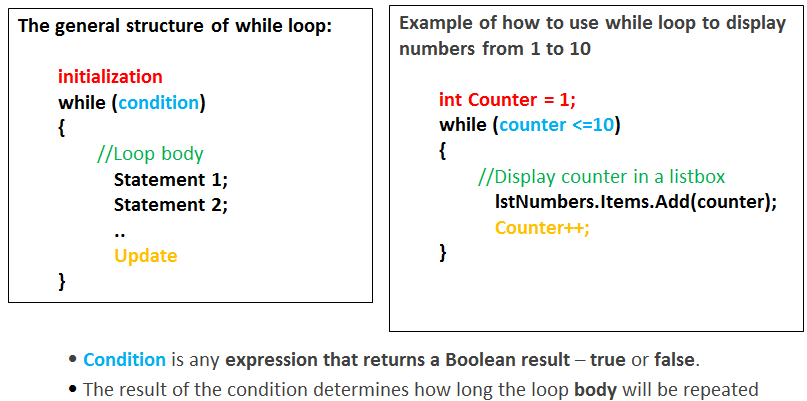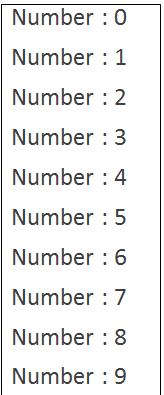# Loops in C#

### What is an iteration?

In programming some problems require the repetition of some instructions.
A loop is a basic programming construct that allows repeated execution of instructions.
In this course, we will focus on while and for loops.

#### While Loop

One of the simplest and most commonly used loops is the "while" loop:You must specify the three actions:

• Initialization: a one-time expression that defines the initial value of the counter. Before the start of the while loop.
• Condition: A Boolean expression to be tested. If true, the loop iterates.
• Update: increase or decrease the value of the counter, in the body of the while loop:#### Example

Write an algorithm/pseudocode for an application that uses a while loop display numbers from 0 to 9 in a List-box.

• Set counter to 0
• while counter <= 9
• Display counter
• counter = counter + 1

##### The C# code for this example is as follows:
```		// Declare & Initialize the counter
int counter = 0;
// Execute the loop body while the loop condition is true
while (counter <= 9)
{
lstNumbers.Items.Add("Number : " + counter);  // Display the counter value
counter++; 	// Increase the counter by 1
}
```
Here is how the output looks like: